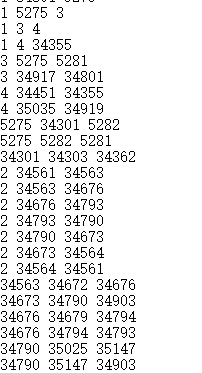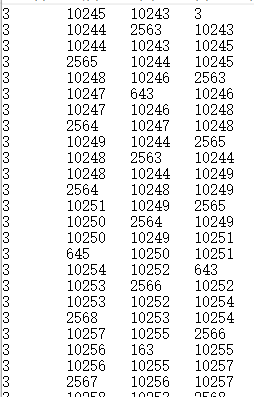• 如何矩阵增行增列
千次阅读
2018-01-22 08:48:15

1、矩阵中添加行：

numpy.row_stack(mat, a)

2、矩阵中添加列：

numpy.column_stack(mat,a)

#coding:utf-8
import numpy as np
#一维向量必须reshape为规范形式
#添加行，列数必须相同
#结果是4行3列
a=np.array([[1,2,3],[4,5,6]])
b=np.array([[1,2,3],[4,5,6]])
print np.row_stack((a,b))
#添加列，行数必须相同
#[1,2,3]  [4,5]--->  [1,2,3,4,5]
a=np.array([1,2,3]).reshape(1,3)
b=np.array([4,5]).reshape(1,2)
print np.column_stack((a,b))

更多相关内容
• 关于matlab矩阵的基本用法：矩阵增加一或一，在实际程序操作中十分有用。
• 如何给 numpy 数据， 指定插入一或者一数据， insert 还是相当好玩的， 可以指定位置插入，相对于其他的还是挺强大的。 添加一数据： 方法1: 使用 insert c = np.array([[[1,2,3],[4,5,6]],[[7,8,9],...

# 添加一列数据：

### 方法1: 使用 insert

c = np.array([[[1,2,3],[4,5,6]],[[7,8,9],[10,11,12]]])
mylist = []
for i in c:
f = np.insert(i, 0, np.array([10, 20]), axis=1)
mylist.append(f)
arr1 = np.array(mylist)
# print(arr1)

结果:
[[[10  1  2  3]
[20  4  5  6]]

[[10  7  8  9]
[20 10 11 12]]]


### 方法2： 使用insert  + 列表生成式   可以插入到指定的位置中去

c = np.array([[[1,2,3],[4,5,6]],[[7,8,9],[10,11,12]]])
arr2 = np.array([np.insert(i, 0, np.array([10, 20]), axis=1) for i in c])
print(arr2)

结果:
[[[10  1  2  3]
[20  4  5  6]]

[[10  7  8  9]
[20 10 11 12]]]

### 方法3: np.c_[a,b]  函数

c = np.array([[[1,2,3],[4,5,6]],[[7,8,9],[10,11,12]]])
d = np.array([100, 200])
mylist = []
for i in c:
q = np.c_[i, d]
mylist.append(q)
arr3 = np.array(mylist)
print(arr3)

结果:
[[[  1   2   3 100]
[  4   5   6 200]]

[[  7   8   9 100]
[ 10  11  12 200]]]

### 方法4: np.c_[a,b]  函数 + 列表生成式


c = np.array([[[1,2,3],[4,5,6]],[[7,8,9],[10,11,12]]])
d = np.array([100, 200])
arr4 = np.array([np.c_[i, d].tolist() for i in c])
print(arr4)

结果:
[[[  1   2   3 100]
[  4   5   6 200]]

[[  7   8   9 100]
[ 10  11  12 200]]]


### 方法5: column_stack 函数 + 列表生成式

c = np.array([[[1,2,3],[4,5,6]],[[7,8,9],[10,11,12]]])
d = np.array([100, 200])
arr5 = np.array([np.column_stack((i,d)) for i in c])
print(arr5)

结果:
[[[  1   2   3 100]
[  4   5   6 200]]

[[  7   8   9 100]
[ 10  11  12 200]]]

# 添加一行数据:

### 方法一: 加一行  np.row_stack 函数   添加行

ls = []
c = np.array([[[1,2,3],[4,5,6]],[[7,8,9],[10,11,12]]])
d = np.array([100, 200, 300])
for i in c:
q = np.row_stack((i, d))
ls.append(q)
arr7 = np.array(ls)
print(arr7)

结果:
[[[  1   2   3]
[  4   5   6]
[100 200 300]]

[[  7   8   9]
[ 10  11  12]
[100 200 300]]]

### 方法2 ：使用 insert 语法 添加行

ls = []
c = np.array([[[1,2,3],[4,5,6]],[[7,8,9],[10,11,12]]])
d = np.array([100, 200, 300])
for i in c:
q = np.insert(i, 0, np.array([100, 200, 300]), axis=0)
ls.append(q)
arr8 = np.array(ls)
print(arr8)

结果:
[[[  1   2   3]
[  4   5   6]
[100 200 300]]
[[  7   8   9]
[ 10  11  12]
[100 200 300]]]

展开全文• Matlab 在矩阵前增加一并将其保存至txt前言：最近在做数据处理时候，想在之前的txt文件前增加一数字，遂coding了此代码！文件处理前(选取其中一段)：文件处理后：Matlab 代码：%% 清除数据和命令行clear all;clc...

Matlab 在矩阵前增加一列并将其保存至txt

前言：最近在做数据处理时候，想在之前的txt文件前增加一列数字，遂coding了此代码！

文件处理前(选取其中一段)：文件处理后：Matlab 代码：%% 清除数据和命令行

clear all;

clc;

%% 加载文件并将其赋值给矩阵

%% 将matrixA矩阵前增加一列3

row=size(matrixA,1); % get row

matrixB=zeros(row,1);

matrixB(:)=3;

matrixA=[matrixB,matrixA];

%% 将matrixA保存到 *.txt 文件中

fid=fopen('File2.txt','wt');%写入文件路径

matrix=matrixA %input_matrix为待输出矩阵

[m,n]=size(matrix);

for i=1:1:m

for j=1:1:n

if j==n

fprintf(fid,'%g\n',matrix(i,j));

else

fprintf(fid,'%g\t',matrix(i,j));

end

end

end

fclose(fid);

展开全文• ## Eigen 矩阵增加一列

千次阅读 2020-08-14 19:08:52
目前想实现在矩阵后添加一的功能，如 A=[1 2 3; 4 5 6] B=[1 2 3] A=[A;B] 在eigen中可以使用repmat定义一个新的临时矩阵，然后将A,B放进去 但是我想要像matlab一样简单，百度不到，google了一下 ...

目前想实现在矩阵后添加一行的功能，如

A=[1 2 3;
4 5 6]
B=[1 2 3]
A=[A;B]


在eigen中可以使用repmat定义一个新的临时矩阵，然后将A,B放进去

https://stackoverflow.com/questions/27404811/append-column-to-matrix-using-eigen-library

使用conservativeResize函数

mat.conservativeResize(mat.rows(), mat.cols() + 1);
mat.col(mat.cols() - 1) = vec;


直接使原函数增加一行，填充即可

展开全文• 在学习中经常会遇到合并矩阵的情况，有时需要在行上合并，有时需要在上合并。具体方法如下： 1、首先随机生成两个矩阵 a = np.floor(10np.random.rand(2,2)) b = np.floor(10np.random.rand(2,2)) 2、在行上...python
• 目录opencv 二维mat矩阵增加一用途代码输出结果参考完 opencv 二维mat矩阵增加一 用途 有时候需要让矩阵增加一，遇上的实例是我想把求得的仿射矩阵(2x3)变成一个透视变换矩阵(3x3)方便后面的运算，只需要增加{...
• 3.4　增加或删除矩阵 严格来说，矩阵的长度和维度是固定的，因此不能增加或删除。但是可以给矩阵重新赋值，这样可以得到和增加或删除一样的效果。3.4.1　改变矩阵的大小回忆之前通过重新赋值改变向量...
• https://www.zybang.com/question/277b5a314c28e200f90cfd721b42a596.html
• 0 9 7]这是一个3*3矩阵，那么当你想插入一r=[1 2 3]时，那么可以这样做：A=[A;r]这样A就变成了4*3的矩阵A如下：A=[2 3 5;3 4 1;0 9 7;1 2 3]假如你已有矩阵A为：A=[2 3 5;3 4 1;0 9 7;1 2 3]那...答：用A...
• ## python 矩阵增加一行或一列

万次阅读 多人点赞 2017-04-05 10:47:16
矩阵增加np.row_stack() 与 np.column_stack()“ import numpy as npa = np.array([[4, 4,], [5, 5]])c = np.row_stack((a, [8,9])) d = np.column_stack((a, [8,9])) “python
• 转载：...加一 A=[1 2 3;4 5 6;7 8 9]; row=[0 0 0]; ...加一 A=[1 2 3;4 5 6;7 8 9]; column=[0;0;0]; %一共三，用“;”分开 A_column=[A column]; display(A_column); ...
•python
• 由于python本身就是动态语言，因此，若想向已经创建好的矩阵中，在增加一或一的话，可以使用numpy中的row_stack以及column_stack函数。 向矩阵中增加一： 先创建如下矩阵，即生成一个3*3的单位矩阵 oadta = ...python
• %最后一添加一个元素。。 s=[]; for i=1:6 if i>2 s=[s;i]; end end s %最后一添加一整行 s1=[]; A=reshape([1:16],4,4);...%添加一，该只有一个元素。 s3=[]; for i=1:4 s3=[s3,i]; end ...matlab
• 严格来说，矩阵的长度和维度是固定的，因此不能增加或删除。但是可以给矩阵重新赋值，这样可以得到和增加或删除一样的效果。    函数rbind()（代表row bind，按组合）和函数cbind()（代表column bind...R语言
• ## Numpy中如何给矩阵增加一行或一列

万次阅读 多人点赞 2016-03-06 16:27:55
使用Python的numpy的array结构，如何给矩阵增加一或者一呢？ 下面提供一种方法，当然numpy还提供了很多API函数可供选择。python
• np.insert的第二个参数是插入的位置，axis用来控制是插入还是，可见该方法非常灵活！ 个人比较推荐使用这个方法 方法3 import numpy as np a = np.array([[1,2,3],[4,5,6],[7,8,9]]) b = np....
• 输入为矩阵 插入一整行 import numpy as np a = a = np.array([[1, 1], [2, 2], [3, 3]]) print('a=',a) print('after insertion:\n',np.insert(a,,[6,6],axis=0)) 结果 a= [[1 1] [2 2] [3 3]] after insertion...python 机器学习 数据结构
• Matlab中怎么给大小未知的矩阵添加一或者一相同元素？ 要想解决这个问题，首先要了解两个函数 size() size函数用来获取矩阵的大小，假如有未知大小的矩阵A [m,n] = size(A);可以通过返回的m知道矩阵A的行数，...
• 矩阵中添加： numpy.row_stack(mat, a) 其中a是要加入的序列 矩阵中添加： numpy.column_stack(mat,a) 其中a是要加入的序列
• M1=[1;1;1;1;1;25;0;0;0;0;0;0;0;0;0;0;0;0;0;0;0;0;0;0;0;0]I=[0000000000000000000011111;%1000000000000000000011...
• 有时我们需要将矩阵 m×n×p 转化为 m×n×p×1 以实现多维矩阵叠加的效果，操作十分简单： import numpy as np A = np.array([ A ]) // A为待操作矩阵
• 矩阵的合并、矩阵大小的改变。矩阵某一行列的单独删除、重新赋值，矩阵元素的访问matlab
• 需要注意的是：matlab计算协方差的函数cov，是按表示维度，减去每均值然后计算协方差矩阵的。 load ( 'hald' , 'ingredients' ) ingredients = ingredients' ; test = cov ( ingredients ) ......